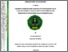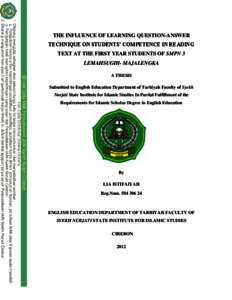# THE INFLUENCE OF LEARNING QUESTION-ANSWER TECHNIQUE ON STUDENTS’ COMPETENCE IN READING TEXT AT THE FIRST YEAR STUDENTS OF SMPN 3 LEMAHSUGIH- MAJALENGKA

LIA ISTIFAIYAH, (2012) THE INFLUENCE OF LEARNING QUESTION-ANSWER TECHNIQUE ON STUDENTS’ COMPETENCE IN READING TEXT AT THE FIRST YEAR STUDENTS OF SMPN 3 LEMAHSUGIH- MAJALENGKA. Bachelor thesis, IAIN Syekh Nurjati Cirebon.Preview
Text
LIA ISTIFAIYAH_58430624__ok.pdf

Download (1MB) | Preview

## Abstract

LIA ISTIFAIYAH : Reg. Num. 58430624 “THE INFLUENCE OF LEARNING QUESTION-ANSWER TECHNIQUE ON STUDENTS’ COMPETENCE IN READING TEXT AT THE FIRST YEAR STUDENTS OF SMPN 3 LEMAHSUGIH-MAJALENGKA” Question-answer technique is one of some techniques in teaching and learning especially in reading text comprehension. The students demanded to be able to read and comprehend the content of the reading texts. But in English lesson, especially in reading comprehension at SMPN 3 Lemahsugih-Majalengka, they still have many problems that must be overcome. Most of the students feel difficult to understand the content of the reading texts and become passive in learning. The aims in this research are to know the application of learning question-answer technique become more active to the students, to know the students’ competence in reading text and to know the positive and significant influence of learning question-answer technique on students’ competence in reading text at the first year students of SMPN 3 Lemahsugih-Majalengka. In this research, the method used is a quantitative one. The place of the research in SMPN 3 Lemahsugih-Majalengka and began to do research on Mei-July 2012. Because the population at the first year students of SMPN 3 Lemahsugih-Majalengka more than 100, so the researcher take 25% from 164 (total population) are 41 students. To know the students’ competence in learning question-answer technique, the writer presented the test to students and to find out the quantitative data of result of test on reading by the students who are taught with question-answer technique, the writer used the formula of Product Moment by Pearson to analysis the data and T count formula to know the hypothesis of this researcher is weather refused or accepted. The result of correlation coefficient between the Learning question-answer technique and the students’ competence in reading text is 0.88 has positive correlation. The value of rxy is 0.88 > 0.304 or rxy > rt. It means that the value is bigger than value in the table at significant degree 5%, with value of DC is 77%. The value of “t” with df = N-2 or 41- 2 = 39 is 11.43 and the value of “t” distribution in significant 5% is 2.02. So, the value of “t” is bigger than “t” distribution in table (t count > t table), it means significant value. So, Ha is accepted and Ho is rejected. In order to word, there is positive and significant correlation between the learning question-answer technique on students’ competence in reading text at SMPN 3 Lemahsugih-Majalengka 2012-2013 with correlation coefficient value is 0.88, it means that is high correlation, because it’s between 0.71-0.90.

Item Type: Thesis (Bachelor) Pendidikan > Pendidikan (Umum) tuti alawiyah alawiyah 22 Mar 2017 01:47 06 Jun 2017 06:17 http://repository.syekhnurjati.ac.id/id/eprint/1210

### Actions (login required)View Item